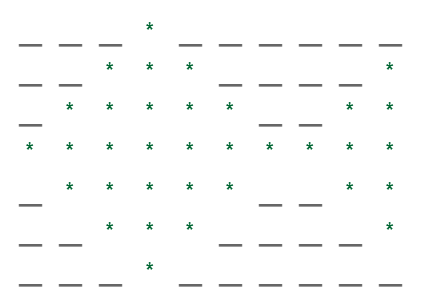# Program to print the Fish Pattern

• Difficulty Level : Basic
• Last Updated : 23 Nov, 2021

Given an integer N, the task is to print a pattern of fish over 2N+1 rows.

Example:

Input: N=3
Output:
*
***    *
*****  **
**********
*****  **
***    *
*

Input: N=5
Output:
*
***        *
*****      **
*******    ***
*********  ****
****************
*********  ****
*******    ***
*****      **
***        *
*

Approach: The fish consists of three parts:

• Upper Part: Over N rows.
• Middle Part: Singe row in the middle
• Lower Part: Over N rows

Now, let’s try to understand the pattern using an example:
For N=3, the fish is:Now, to solve this question, follow the steps below:

1. First, create the upper part:
• Run a loop for i=0 to i<N, and in each iteration of the loop:
• As depicted in the above image that first a string, say spaces1 having M (initially M=N) spaces appear, then a layer of stars, let’s say stars1 having only 1 star, then the string spaces1 appears 2 times (having 2*M spaces) and then another layer of stars, say stars2 having 0 stars initially.
• Now in each iteration, spaces1 got reduced by a space, stars1 got increased by 2 stars and stars2 by 1 star.
2. For the middle part, just print both stars1 and stars2, as no spaces appear in this row.
3. Now, to get the lower part, reverse the algorithm for the upper part.
4. After the loop ends, the pattern of fish will be created.

Below is the implementation of the above approach.

## C++

 `// C++ program for the above approach` `#include ``using` `namespace` `std;` `// Function to print the pattern of a fish``// over N rows``void` `printFish(``int` `N)``{` `    ``string spaces1 = ``""``, spaces2 = ``""``;``    ``string stars1 = ``"*"``, stars2 = ``""``;``    ``for` `(``int` `i = 0; i < N; ++i) {``        ``spaces1 += ``' '``;``    ``}``    ``spaces2 = spaces1;` `    ``for` `(``int` `i = 0; i < 2 * N + 1; ++i) {``        ``// For upper part``        ``if` `(i < N) {``            ``cout << spaces1 << stars1``                 ``<< spaces1 << spaces1``                 ``<< stars2 << endl;``            ``spaces1.pop_back();``            ``stars1 += ``"**"``;``            ``stars2 += ``"*"``;``        ``}` `        ``// For middle part``        ``if` `(i == N) {``            ``cout << spaces1 << stars1``                 ``<< spaces1 << spaces1``                 ``<< stars2 << endl;``        ``}` `        ``// For lower part``        ``if` `(i > N) {``            ``spaces1 += ``' '``;``            ``stars1.pop_back();``            ``stars1.pop_back();``            ``stars2.pop_back();``            ``cout << spaces1 << stars1``                 ``<< spaces1 << spaces1``                 ``<< stars2 << endl;``        ``}``    ``}``}` `// Driver Code``int` `main()``{``    ``int` `N = 5;``    ``printFish(N);``}`

## Java

 `// Java program for the above approach``class` `GFG {` `    ``// Function to print the pattern of a fish``    ``// over N rows``    ``public` `static` `void` `printFish(``int` `N) {` `        ``String spaces1 = ``""``;``        ``String stars1 = ``"*"``, stars2 = ``""``;``        ``for` `(``int` `i = ``0``; i < N; ++i) {``            ``spaces1 += ``' '``;``        ``}` `        ``for` `(``int` `i = ``0``; i < ``2` `* N + ``1``; ++i)``        ``{``          ` `            ``// For upper part``            ``if` `(i < N) {``                ``System.out.print(spaces1 + stars1 + spaces1 + spaces1);``                ``System.out.println(stars2);``                ``spaces1 = spaces1.substring(``0``, spaces1.length() - ``1``);``                ``stars1 += ``"**"``;``                ``stars2 += ``"*"``;``            ``}` `            ``// For middle part``            ``if` `(i == N) {``                ``System.out.print(spaces1 + stars1 + spaces1 + spaces1);``                ``System.out.println(stars2);``            ``}` `            ``// For lower part``            ``if` `(i > N) {``                ``spaces1 += ``' '``;``                ``stars1 = stars1.substring(``0``, stars1.length() - ``1``);``                ``stars1 = stars1.substring(``0``, stars1.length() - ``1``);``                ``stars2 = stars2.substring(``0``, stars2.length() - ``1``);``                ``System.out.print(spaces1 + stars1 + spaces1 + spaces1);``                ``System.out.println(stars2);``            ``}``        ``}``    ``}` `    ``// Driver Code``    ``public` `static` `void` `main(String args[]) {``        ``int` `N = ``5``;``        ``printFish(N);``    ``}``}` `// This code is contributed by gfgking.`

## Python3

 `# Python3 program for the above approach` `# Function to print the pattern of a fish``# over N rows``def` `printFish(N) :` `    ``spaces1 ``=` `"``"; spaces2 = "``";``    ``stars1 ``=` `"*"``; stars2 ``=` `"";``    ``for` `i ``in` `range``(N) :``        ``spaces1 ``+``=` `' '``;` `    ``spaces2 ``=` `spaces1;` `    ``for` `i ``in` `range``( ``2` `*` `N ``+` `1``) :``        ``# For upper part``        ``if` `(i < N) :``            ``print``(spaces1,end``=``"");``            ``print``(stars1,end``=``"");``            ``print``(spaces1,end``=``"");``            ``print``(spaces1,end``=``"");``            ``print``(stars2);``            ``spaces1 ``=` `spaces1[:``-``1``]``            ``stars1 ``+``=` `"**"``;``            ``stars2 ``+``=` `"*"``;` `        ``# For middle part``        ``if` `(i ``=``=` `N) :``            ``print``(spaces1,end``=``"");``            ``print``(stars1,end``=``"");``            ``print``(spaces1,end``=``"");``            ``print``(spaces1,end``=``"");``            ``print``(stars2);` `        ``# For lower part``        ``if` `(i > N) :``            ``spaces1 ``+``=` `' '``;``            ``stars1 ``=` `stars1[:``-``1``];``            ``stars1 ``=` `stars1[:``-``1``];``            ``stars2 ``=` `stars2[:``-``1``];``            ` `            ``print``(spaces1,end``=``"");``            ``print``(stars1,end``=``"")``            ``print``(spaces1,end``=``"");``            ``print``(spaces1,end``=``"");``            ``print``(stars2);`` ` `# Driver Code``if` `__name__ ``=``=` `"__main__"` `:` `    ``N ``=` `5``;``    ``printFish(N);` `    ``# This code is contributed by AnkThon`

## C#

 `// C# program for the above approach``using` `System;``class` `GFG {` `    ``// Function to print the pattern of a fish``    ``// over N rows``    ``static` `void` `printFish(``int` `N) {` `        ``string` `spaces1 = ``""``;``        ``string` `stars1 = ``"*"``, stars2 = ``""``;``        ``for` `(``int` `i = 0; i < N; ++i) {``            ``spaces1 += ``' '``;``        ``}` `        ``for` `(``int` `i = 0; i < 2 * N + 1; ++i)``        ``{``          ` `            ``// For upper part``            ``if` `(i < N) {``                ``Console.Write(spaces1 + stars1 + spaces1 + spaces1);``                ``Console.Write(stars2 + ``"\n"``);``                ``spaces1 = spaces1.Substring(0, spaces1.Length - 1);``                ``stars1 += ``"**"``;``                ``stars2 += ``"*"``;``            ``}` `            ``// For middle part``            ``if` `(i == N) {``                ``Console.Write(spaces1 + stars1 + spaces1 + spaces1);``                ``Console.Write(stars2 + ``"\n"``);``            ``}` `            ``// For lower part``            ``if` `(i > N) {``                ``spaces1 += ``' '``;``                ``stars1 = stars1.Substring(0, stars1.Length - 1);``                ``stars1 = stars1.Substring(0, stars1.Length - 1);``                ``stars2 = stars2.Substring(0, stars2.Length - 1);``                ``Console.Write(spaces1 + stars1 + spaces1 + spaces1);``                ``Console.Write(stars2 + ``"\n"``);``            ``}``        ``}``    ``}` `    ``// Driver Code``    ``public` `static` `void` `Main() {``        ``int` `N = 5;``        ``printFish(N);``    ``}``}` `// This code is contributed by Samim Hossain Mondal.`

## Javascript

 ``

Output

```     *
***        *
*****      **
*******    ***
*********  ****
****************
*********  ****
*******    ***
*****      **
***        *
*          ```

Time Complexity: O(N)
Auxiliary Space: O(N)

My Personal Notes arrow_drop_up